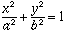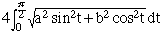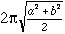What is the formula for the perimeter or circumference of an ellipse? Hi Kurtis, There is no simple formula for the perimeter of an ellipse. The exact value is given by an ELLIPTIC INTEGRAL OF THE SECOND TYPE -- in the past people used extensive tables to find approximate answers, but today one gets greater accuracy using a calculator to approximate the integral. When the ellipse has semi-axes of length a and b (so that its equation isthe perimeter equalsA simple approximate value isCheers, Chris Go to Math Central